# Algebra II : Adding and Subtracting Fractions

## Example Questions

### Example Question #4371 : Algebra Ii

Simplify: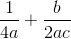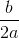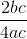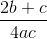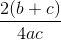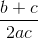Explanation:

To add or subtract fractions, the fractions must have the same denominator.

The smallest common denominator in this case is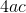.

After multiply both terms so that they have the same denominator.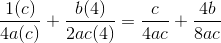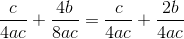Simply add these two terms together.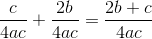### Example Question #41 : Fractions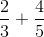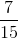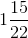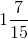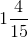Explanation:

To solve this we need to first find common denominators. We do that by multiplying the first fraction by 5 on the top and bottom and the second fraction by 3 on the top and bottom.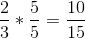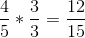Add these fractions together, then write it as a mixed number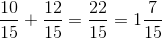### Example Question #42 : Fractions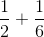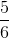Explanation:

To solve this we need to first find common denominators. We do that by multiplying the first fraction by 3 over 3.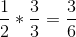Add these fractions together, then reduce it to get the final answer.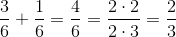### Example Question #43 : Fractions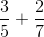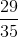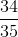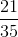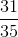Explanation:

To solve this we need to first find common denominators. We do that by multiplying the first fraction by 7 over 7 and the second fraction by 5 over 5.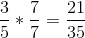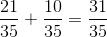### Example Question #7 : Subtraction With Fractions

Subtract these fractions: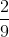Explanation:

To solve this we need to first find common denominators. We do that by multiplying the first fraction by 2 over 2 and the second fraction by 3 over 3.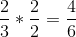Subtract these fractions to get the final answer.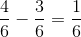### Example Question #11 : Adding And Subtracting Fractions

Subtract these fractions: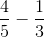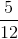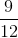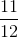Explanation:

To solve this we need to first find common denominators. We do that by multiplying the first fraction by 3 over 3 and the second fraction by 5 over 5.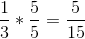Subtract the numerators of the fractions to get the final answer.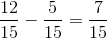### Example Question #31 : Operations With Fractions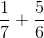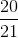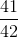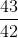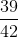Explanation:

To solve this we need to first find common denominators. We do that by multiplying the first fraction by 6 over 6 and the second fraction by 7 over 7.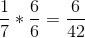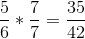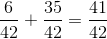### Example Question #45 : Fractions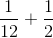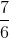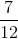Explanation:

To solve this we need to first find common denominators. We do that by multiplying the second fraction by 6 over 6.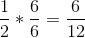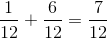### Example Question #46 : Fractions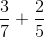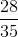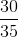Explanation:

To solve this we need to first find common denominators. We do that by multiplying the first fraction by 5 over 5 and the second fraction by 7 over 7.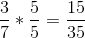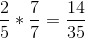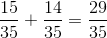### Example Question #47 : Fractions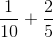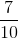Explanation:

To solve this we need to first find common denominators. We do that by multiplying the second fraction by 2 over 2.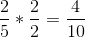Add the numerators of these fractions and simplify to get the final answer.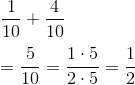### All Algebra II Resources